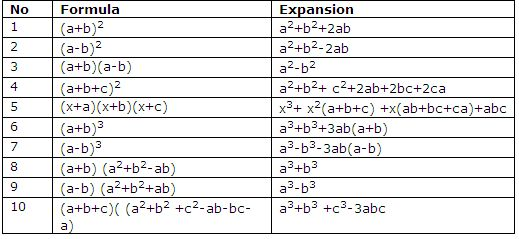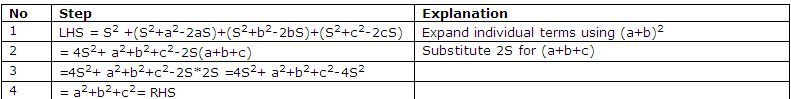Name: ___________________Date:___________________

 Email us to get an instant 20% discount on highly effective K-12 Math & English kwizNET Programs!

### High School Mathematics - 25.1 Conditional IdentitiesExample: If a+b+c = 2S prove that S2+(S-a)2+ (S-b)2+(S-c)2= a2+b2+c2Directions: Prove the following.Name: ___________________Date:___________________

### High School Mathematics - 25.1 Conditional Identities

 Q 1: If a+b+c 0 and a3+b3+c3 =3abc prove that a=b=c Answer: Q 2: If a+b+c = 2S prove that a2+b2- c2+2ab/ a2-b2+ c2+2ac = (S-c)/(S-b) Answer: Question 3: This question is available to subscribers only! Question 4: This question is available to subscribers only!